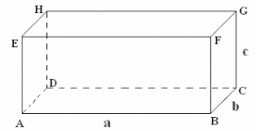The edge lengths of a quadrilateral prism are in the ratio a: b: c = 2: 4: 5. The surface of the prism is 57 cm2. Calculate the volume.

V =  25.9808 cm3

### Step-by-step explanation:Did you find an error or inaccuracy? Feel free to write us. Thank you!

Tips for related online calculators
Are you looking for help with calculating roots of a quadratic equation?
Need help calculating sum, simplifying, or multiplying fractions? Try our fraction calculator.
Check out our ratio calculator.
Do you have a linear equation or system of equations and looking for its solution? Or do you have a quadratic equation?